This mathematics ClipArt gallery offers 127 images that can be used to demonstrate various geometric theorems and proofs.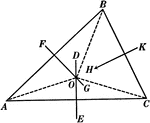### Perpendicular Bisectors In A Triangle

Illustration used to prove "The perpendicular bisectors of the sides of a triangle are concurrent in…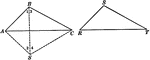### Equal Triangles

Illustration used to show that two triangles are equal if the three sides of one are equal respectively…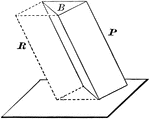### Volume of Triangular Prism

Diagram used to prove the theorem: "The volume of a triangular prism is equal to the product of its…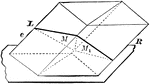### Parallelopiped Divided Into Triangular Prisms

Diagram used to prove the theorem: "The plane passed through two diagonally opposite edges of a parallelopiped…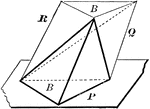### Volume of Triangular Pyramid

Diagram used to prove the theorem: "The volume of a triangular pyramid is equal to one third of a triangular…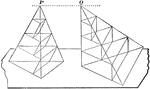### Equivalent Triangular Pyramids

Diagram used to prove the theorem: "Two triangular pyramids having equivalent bases and equal altitudes…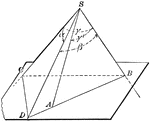### Face Angles of Trihedral Angle

Diagram used to prove the theorem: "The sum of two face angles of a trihedral angle is greater than…